• landsat 5-8不同传感器的地表温度反演模型以及参数设置单窗算法
• Landsat 8地表温度反演免费软件，Landsat 8地表温度反演免费软件，北京大学团队研究开发的，免费下载哈，有需要的可以看一下。
• landsat 8 地表温度反演 ，操作简单，自带使用说明。
• 常用ENVI功能扩展工具之一：Landsat8LST-地表温度反演ENVI
• ## 地表温度反演操作总结

万次阅读 多人点赞 2019-02-14 16:26:00
地表温度反演操作总结 由于博主也是自己边做实验便总结，难免会有混乱出错的地方，欢迎大家指正~ 整体实验流程： 1 数据预处理 1.1 数据下载 本实验使用的是Landsat8 OLI_TIRS数据，在地理空间数据云...
地表温度反演操作总结
由于博主也是自己边做实验便总结，难免会有混乱出错的地方，欢迎大家指正~
整体实验流程：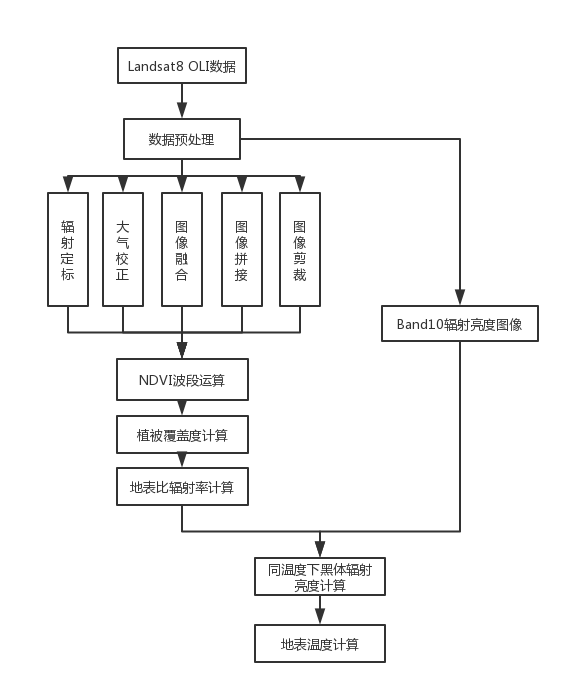1 数据预处理
1.1 数据下载
本实验使用的是Landsat8 OLI_TIRS数据，在地理空间数据云（http://www.gscloud.cn/） 下载三幅广州地区的影像预备进行预处理。（由于这仅为一个练手实验，所以并未对云量有太高要求）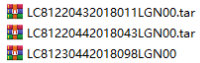1.2 辐射定标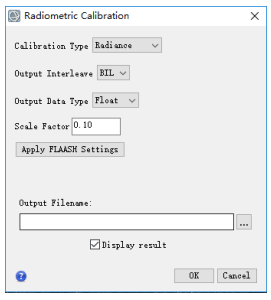1.3 大气校正（温度反演可省去此步，对结果影响不大）
大气校正模型有两种，这里我们使用FLAASH大气校正，打开Radiometric Correction-Atmospheric Correcrion Module-FLAASH Atmospheric Correction工具，打开已定标的多光谱图像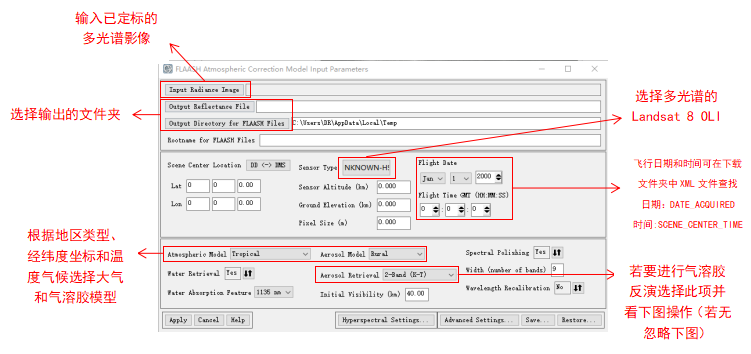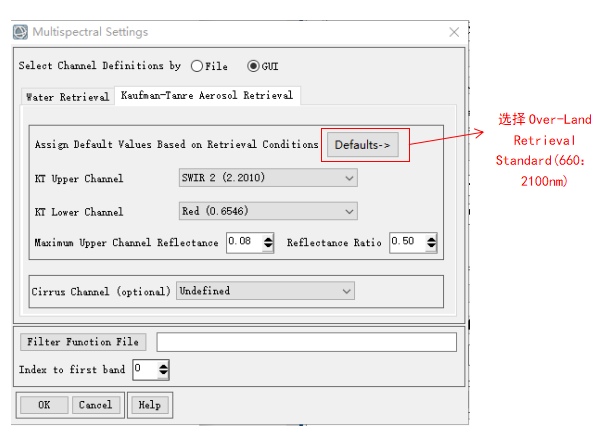1.4 图像融合
使用Image Sharpening-Gam-Schmidt Pan Sharpening工具进行图像融合，先选择低分辨率的多光谱影像（MultiSpectral）后选择高分辨率的全色影像（Panchromatic）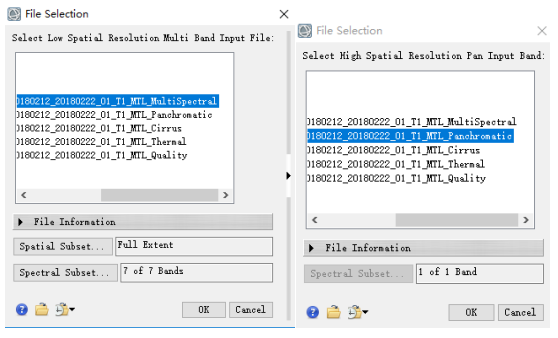选择传感器类型和重采样方法，设置输出文件夹，点击OK输出融合图像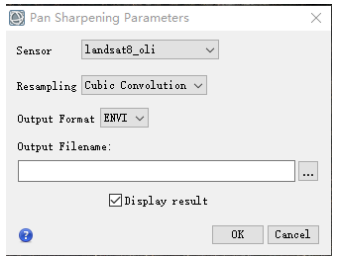1.5 图像拼接
将三幅完成预处理的影像使用 Mosaicking-Seamless Mosaic工具进行拼接
点击加号添加三幅影像：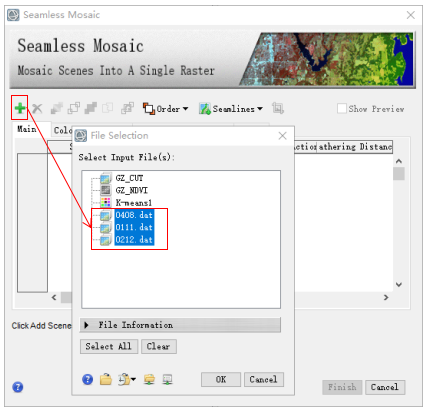Color Matching Action可选择影像颜色的被参照图像和校正图像，选择一副图像为被参照图，另外两副的颜色就会校正成与被参照图相似的颜色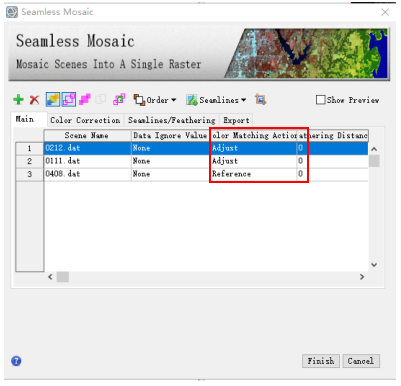勾选Histogram Matching，这里主要是选择颜色改变是交界处改变还是全图改变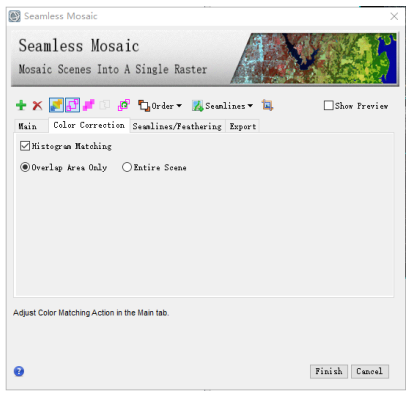选择Auto Generate Seamlines自动生成缝纫线，能观察到如何裁剪图像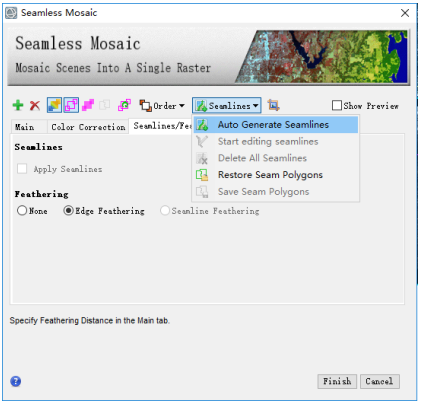设置输出背景，输出文件夹和格式，点击Finish便可得到拼接文件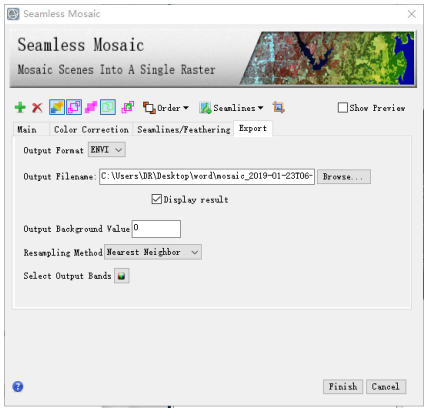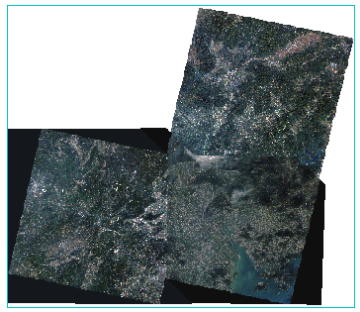注：可勾选右上角Show Preview观察每一步操作后的图像
1.6 图像裁剪
现在我们得到三幅影像拼接的图像，我们要准确的切割出广州市的影像
打开广州市边界矢量图，使用Regions of Interest-Vector to ROI工具将矢量图转化为ROI方便切割（注意：若是两个坐标系不一致，还需要进行投影变换）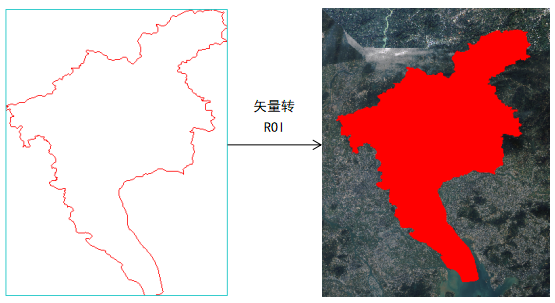使用Subset Data from ROIs工具进行裁剪
先选择拼接好的图像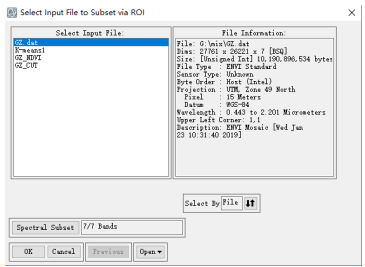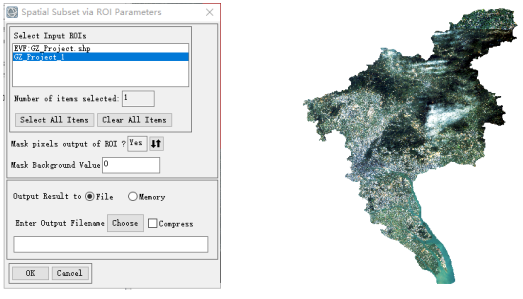1.7 Band10辐射亮度图像获取
Band10波段后缀为Thermal，打开后照以上步骤拼接剪裁，然后进行辐射定标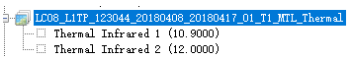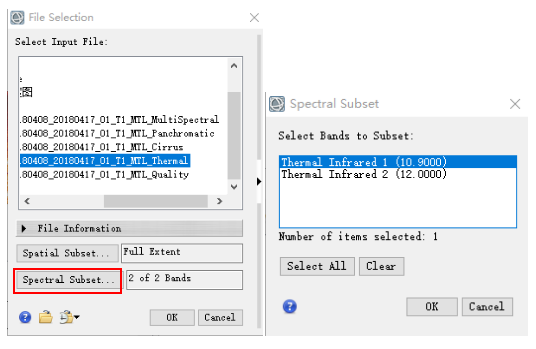然后开始进行辐射定标，设定如下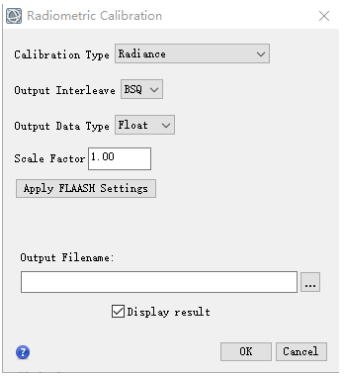2 地表温度反演
本实验采用大气校正法。
大气校正法基本原理：首先估计大气对地表热辐射的影响, 然后把这部分大气影响从卫星传感器所观测到的热辐射总量中减去, 从而得到地表热辐射强度, 再把这一热辐射强度转化为相应的地表温度。
卫星传感器接收到的热红外辐射亮度值Lλ由三部分组成：大气向上辐射亮度L↑，地面的真实辐射亮度经过大气层之后到达卫星传感器的能量；大气向下辐射到达地面后反射的能量。卫星传感器接收到的热红外辐射亮度值Lλ的表达式可写为（辐射传输方程）：
Lλ = [εB(TS) + (1-ε)L↓]τ + L↑
式中，ε为地表比辐射率，TS为地表真实温度(K)，B(TS)为黑体热辐射亮度，τ为大气在热红外波段的透过率。则温度为T的黑体在热红外波段的辐射亮度B(TS)为：
B(TS) = [Lλ - L↑- τ(1-ε)L↓]/τε
Ts可以用普朗克公式的函数获取。
TS = K2/ln(K1/ B(TS)+ 1)
对于TM，K1 =607.76 W/(m2µmsr)，K2 =1260.56K。
对于ETM+，K1=666.09 W/(m2µmsr)，K2 =1282.71K。
对于TIRS Band10，K1= 774.89 W/(m2µmsr)，K2 = 1321.08K。
2.1 NDVI指数运算
使用Band Math计算NDVI指数(或者可以直接使用ENVI自带的计算NDVI指数工具)，指数公式:(近红外-红)/(近红外+红)
Band Math计算结果输出如下：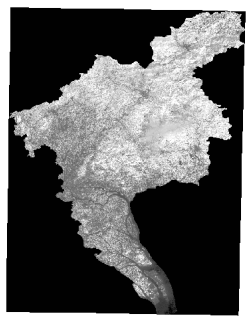2.2 计算植被覆盖度
计算公式：(B1 gt 0.7)*1+(B1 lt 0.05)0+(B1 ge 0.05 and B1 le 0.7)((B1-0.05)/(0.7-0.05))
B1选择NDVI输出结果
Band Math计算结果输出如下：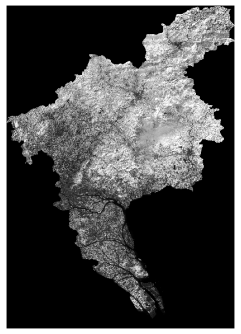2.3 计算地表比辐射率
计算公式：0.004*B1+0.986
B1选择计算植被覆盖度后的结果
Band Math计算结果输出如下：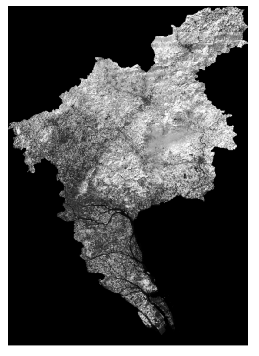2.4计算同温度下的黑体辐射亮度图像
计算公式：(B2-0.75-0.9*(1-B1)1.29)/(0.9B1)
B1选择地表比辐射率图像
B2选Band10辐射亮度图像
Band Math计算结果输出如下：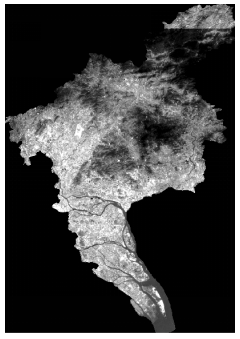2.5计算地表温度
计算公式：(1321.08)/alog(774.89/B1+1)-273
B1选择黑体辐射亮度图像
Band Math计算结果输出如下：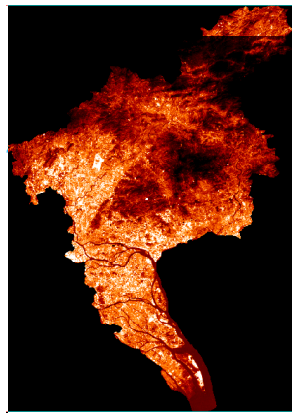使用Cursor Value查看地表温度的数值，大多是在8-15℃的范围内，结合影像拍摄时间为凌晨两点，温度范围合理。


展开全文• 地表温度反演过程，使用软件是ENVI，可以相互交流一下。
• Landsat8地表温度反演单窗算法工具，基于IDL开发，效率高，精度高，简单易用
• PIE-Engine Studio基于影像的通州区地表温度反演.pdf
• 文中介绍了一种基于单通道热红外数据的地表温度反演模型,该模型以大气辐射传输方程为理论推导依据,并利用泰勒展开式可得到求取的众多反演参量。通过对Landsat TM(Thematic Mapper)热波段数据的波谱响应分析,经总结后...
• “基于Modis数据的地表温度反演” 实验过程中需要用到的资料，包含：学生作业、Esri 公开培训资料，网络公开资料等 。配套博客：https://blog.csdn.net/qq_42453941/article/details/106686340#comments_12939738 ...
• 基于Landsat ETM+影像的武威地区地表温度反演与分析，许民，刘勇，陆地表面温度是地-气相互作用及其能量相互交换的综合反映，武威地区地处干旱、半干旱地区，其地表温度（LST）与下垫面覆盖有密切�
• 内含有遥基于遥感数据的地表温度繁衍工具，可用来进行大气校正与单窗算法反演地表温度
• 基于遥感的地表温度反演主要有三种，辐射传输方程法、单窗算法和劈窗算法。在遥感生态指数（RSEI）的地表温度反演用到的是辐射传输方程算法。接下来，简单说一下覃志豪的单窗算法反演地表温度的基本操作。 原理： ...
基于遥感的地表温度反演主要有三种，辐射传输方程法、单窗算法和劈窗算法。在遥感生态指数（RSEI）的地表温度反演用到的是辐射传输方程算法。接下来，简单说一下覃志豪的单窗算法反演地表温度的基本操作。

原理：

其中，Ts为地表真实温度，是我们要计算的值；a、b为常量，a=-67.355351，b=0.458606；C、D为中间变量，为大气平均作用温度（单位：K），可以用普朗克公式的反函数获取。

其中  表示地表比辐射率，t为当天大气的透射率

大气平均作用温度 与近地面气温 （一般为2m）存在以下的线性关系：（注：、的单位均为华氏度 K，而不是摄氏度）

（热带平均大气）

（中纬度夏季平均大气）

（中纬度冬季平均大气）

其中，是大气平均作用温度， 是遥感图像获取时当地的气温。

K1、K2是卫星发射前的预设常量，具体值如下图所示

原理大概就这样。由此，单床算法反演地表温度，需要3个参数，分别是：大气平均作用温度、大气透射率、地表比辐射率。接下来，就是具体操作了~

1、从地理空间空间数据云下载Landsat8卫星遥感影像，尽量找云量少的。本次的研究区是宁夏中卫市沙坡头区。打开头文件，查看该遥感影像的基本信息，如图1所示

3、对多光谱波段进行大气校正，得到可见光波段的反射率图像 （该反射率值被放大大了10000倍)。大气校正参数设置参见文章  遥感生态指数（RSEI)——图像预处理

4、获取研究区当天的大气透射率。计算大气剖面参数的网址：https://atmcorr.gsfc.nasa.gov/

（推荐一个可以查询历史天气的网站：https://www.wunderground.com/

注：该网站气温为华氏度，需换算成摄氏度，大气压单位为英寸汞（inHg），需换算成毫巴），得到结果如图7所示，得到当天的大气透射率为 0.73

5、计算地表比辐射率

①计算NDVI：在bandmath中输入计算归一化植被指数的计算公式 (float(b4-b3))/(b4+b3)   其中，b3、b4分别选择经过大气校正之后的红波段和近红外波段。ndvi的值范围在[0,1]之间，若因为大气校正过度而使ndvi的值超出这个范围，可以使用去除异常值的方法来进行剔除

②计算植被覆盖度Fv：植被覆盖度的计算公式为 Fv = (NDVI - NDVIS)/(NDVIV-NDVIS)  ，其中 NDVIS、NDVIV分别表示NDVI的最小值和最大值。这里，取5%的置信区间，得到NDVIS=0.1，NDVIV = 0.57

因此，在bandmath中输入植被覆盖度度的公式： （b1 gt 0.57)*1+(b1 lt 0.1)*0+(b1 ge 0.1 and b1 le 0.57)*((b1-0.1) / (0.57-01))  ,其中b1为NDVI图像数据

③计算地表比辐射率Surf：根据地物不同，其辐射率也不同，一般将遥感地物分为水体、城镇和自然表面三种。三种不同类型地物像元的比辐射率估算公式如下：

E(water)=0.995

E(surface)=0.9625+0.0614*Fv-0.0461*Fv*Fv

E(building)=0.9589+0.086*Fv-0.0671*Fv*Fv

因此，在bandmath中输入地表比辐射率计算公式： (b1 le 0.1)*0.995+(b1 gt 0.1 and b1 lt 0.57)*(0.9589+0.086*b2-0.0671*b2*b2)+(b1 ge 0.57)*(0.9625+0.0614*b2-0.0461*b2*b2)   其中，b1为NDVI，b2为植被覆盖度Fv，得到地表比辐射率图像 Surf

6、计算中间参数 C

在Bandmath中输入公式 0.73*b1，b1选择地表比辐射率图，即热红外波段辐射校正后的数据，得到 C_2019

7、计算中间参数 D

在Band math中输入计算公式 (1-t)*(1+(1-b1)*t) ,其中，t = 0.73 ，b1选择地表比辐射率图像

8、计算T6 ，即辐射亮温

在Bandmath中输入计算公式 1321.08/alog（774.89/b1+1），b1选择地表比辐射率图像

9、计算Ta（T0的温度要转换成开尔文温度）

由于我的研究区是中纬度夏季，因此，Ta = 16.0110+0.92621*298.705 = 292.67455805  （78°F = 298.705K）

9、计算真实地表温度

在bandmath中输入公式 (-67.355351*(1-b1-b2)+(0.458606*(1-b1-b2)+b1+b2)*b3-b2*292.67455805）/b1  其中，b1、b2、b3分别表示中间参数C、D、T6，计算结果即为单窗算法反演出的地表温度。

若取5%的置信区间，可知沙坡头区2019年8月18日正午12点的地表温度在29-46℃（地表温度比体感温度高~）

10、裁剪研究区、温度分级、出图。效果图如下图所示：（南部的蓝色点点的低温区是由于云层照成的误差~）


展开全文• FY-2G地表温度反演产品改变模式初值对一次台风暴雨模拟的影响研究论文
• 该教程基于辐射传输方程，以landsat遥感影像为数据源，进行地表温度反演。内含的详细步骤讲解和每一步运算的envi 波段计算公式。
• 地表温度作为地球环境分析的重要指标，而遥感技术作为现代重要的对地观测手段，使得基于遥感图像的地表温度反演的研究越来越多。主要的地表温度反演方法有：大气校正法，单窗算法，单通道法等等。本文介绍用辐射传输...
地表温度作为地球环境分析的重要指标，而遥感技术作为现代重要的对地观测手段，使得基于遥感图像的地表温度反演的研究越来越多。主要的地表温度反演方法有：大气校正法，单窗算法，单通道法等等。本文介绍用辐射传输方程法对地表温度进行反演。

 技术流程：


例子数据为2002年9月2日的襄樊市Landsat ETM+数据。根据数据的特点以及地表温度反演研究的技术要求，采用的技术路线为：先对Landsat ETM+数据进行预处理：数据读取、辐射定标、大气校正、襄樊区域裁剪，利用大气校正，即：辐射传输方程法对其影像热红外波段数据进行操作反演，实现襄樊市地区的地表真实温度的反演研究。具体的处理流程如下：

图 1 计算流程

具体的实现步骤如下：

第一步：准备数据

由TM影像（已经过大气校正）生成的NDVI数据，已经利用主菜单->Basic Tools->Resize Data（SFatial/SFectral）重采样为60米分辨率，与TMi6数据保持一致，文件名为：TM-NDVI-60m.img。

第二步：地表比辐射率计算

物体的比辐射率是物体向外辐射电磁波的能力表征。它不仅依赖于地表物体的组成，而且与物体的表面状态(表面粗糙度等)及物理性质(介电常数、含水量等)有关，并随着所测定的波长和观测角度等因素有关。在大尺度上对比辐射率精确测量的难度很大，目前只是基于某些假设获得比辐射率的相对值，本文主要根据可见光和近红外光谱信息来估计比辐射率。

(一)   植被覆盖度计算

计算植被覆盖度Fv采用的是混合像元分解法，将整景影像的地类大致分为水体、植被和建筑，具体的计算公式如下：

FV = (NDVI- NDVIS)／(NDVIV - NDVIS) （2）

其中，NDVI为归一化差异植被指数，取NDVIV = 0.70和NDVIS = 0.00，且有，当某个像元的NDVI大于0.70时，FV取值为1；当NDVI小于0.00，FV取值为0。

利用ENVI主菜单->Basic Tools->Band Math，在公式输入栏中输入：

（b1 gt 0.7）1+（b1 lt 0.）*0+（b1 ge 0 and b1 le 0.7）（(b1-0.0)/(0.7-0.0)）

b1:选择NDVI图像

得到植被盖度图像。

(二)  地表比辐射率计算

根据前人的研究，将遥感影像分为水体、城镇和自然表面3种类型。本专题采取以下方法计算研究区地表比辐射率：水体像元的比辐射率赋值为0.995，自然表面和城镇像元的比辐射率估算则分别根据下式（3）（4）进行计算：

εsurface = 0.9625 + 0.0614FV - 0.0461FV2  （3）

εbuilding = 0.9589 + 0.086FV - 0.0671FV2   （4）

式中，εsurface和εbuilding分别代表自然表面像元和城镇像元的比辐射率。

利用ENVI主菜单->Basic Tools->Band Math，在公式输入栏中输入：

（b1 le 0）0.995+（b1 gt 0 and b1 lt 0.7）(0.9589 + 0.086*b2 - 0.0671*b2^2)+(b1 ge 0.7)*(0.9625 + 0.0614*b2 - 0.0461*b2^2)

b1：NDVI值；

b2：植被覆盖度值。

得到地表比辐射率数据。

第三步：计算相同温度下黑体的辐射亮度值

辐射传输方程法，又称大气校正法，其基本思路为：首先利用与卫星过空时间同步的大气数据来估计大气对地表热辐射的影响。然后把这部分大气影响从卫星高度上传感器所观测到的热辐射总量中减去。从而得到地表热辐射强度．再把这一热辐射强度转化为相应的地表温度.

卫星传感器接收到的热红外辐射亮度值Lλ由三部分组成：大气向上辐射亮度L↑，地面的真实辐射亮度经过大气层之后到达卫星传感器的能量；大气向下辐射到达地面后反射的能量。卫星传感器接收到的热红外辐射亮度值的表达式可写为（辐射传输方程）：

Lλ = [ε·B(TS) + (1-ε)L↓]·τ + L↑  （4）

这里，ε为地表辐射率，TS为地表真实温度，B(TS)为普朗克定律推到得到的黑体在TS的热辐射亮度，τ为大气在热红外波段的透过率。则温度为T的黑体在热红外波段的辐射亮度B(TS)为：

                                             B(TS) = [Lλ - L↑- τ·(1-ε)L↓]/τ·ε    （5）

在NASA官网(http://atmcorr.gsfc.nasa.gov/)中输入成影时间以及中心经纬度，则会提供上式中所需要的参数。本专题输入的数据是襄樊市地区2002年9月2日北京时间10：30成像的Landsat7 ETM+影像，影像中心的经纬度为：32.51N, 111.81 E。得到下图参数图: 大气在热红外波段的透过率τ为0.6，大气向上辐射亮度L↑为3.39 W/(m2·sr·μm)，大气向下辐射亮辐射亮度L↓为5.12W/(m2·sr·μm)。

图2 2002年9月2日Landsat ETM+数据的大气辅助参数


利用ENVI主菜单->Basic Tools->Band Math，在公式输入栏中输入：

（b2-3.39-0.6*（1-b1）*5.12）/（0.6*b1）

b1：60m分辨率的地表比辐射率值；

b2：表示热红外波段大气校正后的辐射定标值。

得到了温度为T的黑体在热红外波段的辐射亮度值。

第四步：反演地表温度

在获取温度为TS的黑体在热红外波段的辐射亮度后，根据普朗克公式的反函数，求得地表真实温度TS：

                      TS = K2/ln(K1/ B(TS)+ 1)


对于ETM+，K1 =666.09W/(m2·sr·μm)，K2 =1282.71K。

利用ENVI主菜单->Basic Tools->Band Math，在公式输入栏中输入：

（1282.71）/alog（666.09/b1 +1）-273

b1：温度为T的黑体在热红外波段的辐射亮度值。

得到真实的地表温度值，单位是摄氏度。

第五步：结果浏览与输出

在DisFlay中显示温度值，是一个灰度的单波段图像。

(1)      选择Tools->Color MaFFing->Density Slice，单击Clear Range按钮清除默认区间。

l  39℃以上，红色

l  35℃至39℃，黄色

l  30℃至35℃，绿色

l   低于30℃，蓝色

(3)      单击Apply。

(4)  选择File->OutFut Range to Class Image，可以将反演结果输出。

图3 地表温度反演结果

地表温度反演结果可以用于很多地方，如城市热岛监测、计算土壤湿度指数（NDVI/T）等。


原文地址：https://blog.csdn.net/esrichinacd/article/details/7534019
展开全文ENVI
• ## 基于Modis数据的地表温度反演

千次阅读 热门讨论 2020-06-11 13:26:19
基于Modis数据的北京市地表温度反演 图片压缩有点看不清 ，留言可发word原文。 操作平台 ENVI 5.5 ArcGIS 10.2 数据源 MODIS B1产品（包含1km 热红外波段） 数据来源 ...研究区：北京市 研究时间：2019年9月 ...
基于Modis数据的北京市地表温度反演
图片压缩有点看不清 ，留言可发word原文。
操作平台
ENVI 5.5
ArcGIS 10.2
数据源
MODIS B1产品（包含1km 热红外波段）
数据来源
研究区：北京市
研究时间：2019年9月
原理介绍
算法：劈窗算法
主要根据覃志豪研究成果进行，针对于陆地
$T_s=A_0+A_1T_{31}-A_2T_{32}$
其中 ${\ T}_s$ 为地表温度，
$A_0、A_1、A_2$ 为参数，
$T_{31}、T_{32}$ 分别为 $B31、B32$ 的亮度温度。
$A_0=\frac{a31D32(1-C31-D31)}{D32C31-D31C32}-\frac{a32D31（1-C32-D32）}{D32C31-D31C32}$
$A_1=1+\frac{D_{31}}{D_{32}C_{31}-D_{31}C_{32}}+\frac{b_{31}D_{32}(1-C_{31}-D_{31})}{D_{32}C_{31}-D_{31}C_{32}}$
$A_2=\frac{D_{31}}{D_{32}C_{31}-D_{31}C_{32}}+\frac{{b_{32}D}_{31}(1-C_{31}-D_{32})}{D_{32}C_{31}-D_{31}C_{32}}$
其中：
$a_{31}=-64.60363，b_{31}=0.440817$
$a_{32}=-68.72575，b_{32}=0.47345$
$C_i$  $D_i$ 均为参数，运算如下
$C_i=\varepsilon_i\tau_i$
$\varepsilon_i$ 为地表比辐射率，$\tau_i$ 为 $\ i$ 热通道大气透过率
$D_i=\left(1-\tau_i\right)[1+(1-\varepsilon_i\tau_i)]$
其中：
地表比辐射率计算
$\varepsilon_i=P_vR_v\varepsilon_{iv}+(1-Pv)Rsεis+dεi$
$P_v$ 为地表植被覆盖度，可以通过归一化植被指数计算
$R_v$ 、$R_s$ 分别为植被和裸土的辐射比率
其中：
$\varepsilon_{31v}=0.98672$
$\varepsilon_{32v}=0.98990$
$\varepsilon_{31s}=0.96767$
$\varepsilon_{32s}=0.97790$
$R_v=0.92762+0.07033P_v$
$R_s=0.99782+0.08362P_v$
$P_v=\frac{NDVI-{NDVI}_S}{{NDVI}_V-{NDVI}_S}$
其中：
${NDVI}_V=0.7$
${NDVI}_S=0.05$
$\ d_\varepsilon=0.003796\min[P_{v\ },(1-P_v)]$
$NDVI=\frac{B_2-B_1}{B_2+B_1}$
其中：
$B_i$ 为第 i 波段的反射率
大气透过率计算
$W=(\alpha-\ln{\frac{{ref}_{19}}{{ref}_2}})/\beta$
其中：
W 为 水汽含量，${ref}_i$  为 I 波段反射率
$\alpha=0.02$
$\beta=0.651$
$y_{31}=5.72-4.69e^{(x/42.05)}$
$y_{32}=\ -1.25+2.28e^{(-x/12.44)}$
亮温计算:
$T_{31}=\frac{K_{31,2}}{\ln{(1+\frac{K_{31,1}}{{Rad}_{31}})}}$
$T_{32}=\frac{K_{32,2}}{\ln{(1+\frac{K_{32,1}}{{Rad}_{32}})}}$
其中：
$T_i$ 为亮温
$K_{31,1}=729.541636$
$K_{31,2}=1304.413871$
$K_{32,1}=474.684780$
$K_{32,2}=1196.978785$
${Rad}_i$ 为I 通道 辐射亮度
操作过程
技术流程图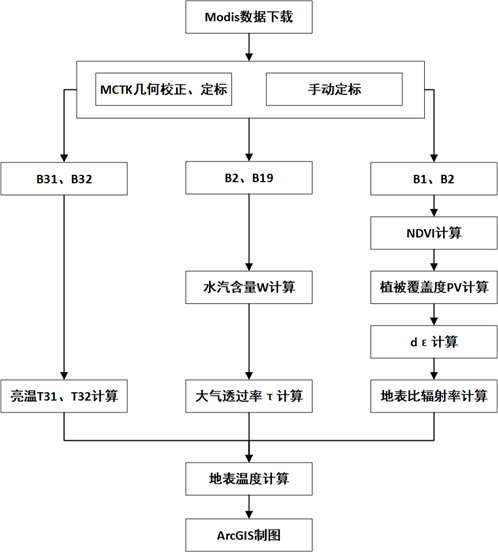具体操作
一、预处理（几何校正与辐射定标）
方法一：MCTK
选用ENVI提供的扩展工具MCTK，进行几何校正，几何校正后的结果同时也进行了定标。首先安装MCTK,然后即可在Toolbox中extensions中找到安装的扩展工具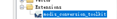打开工具，按照提示输入参数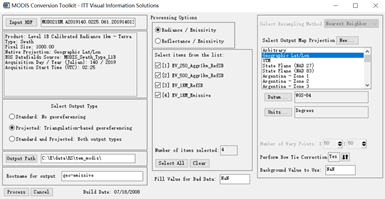方法二：手动定标
通过toolbox中的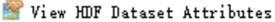工具查看，热红外数据集的scales和 offsets,并通过公式：
$Radiance=scales\times(DN-offsets)$
计算。
使用ENVI中的band math计算出结果
由于后续还需要用到NDVI，所以还需要对B1、B2进行定标。操作相同，不再赘述。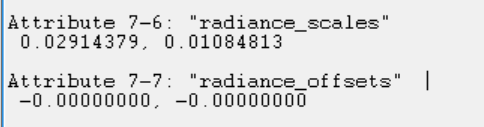结果：MCTTK这种定标方式和手动定标结果有一定出入，所以暂时选择MCTK定标方式。
根据相关研究，做地表温度反演可以不用进行大气校正，所以就不进行大气校正了。
二、计算

计算亮温
计算T31、T32亮温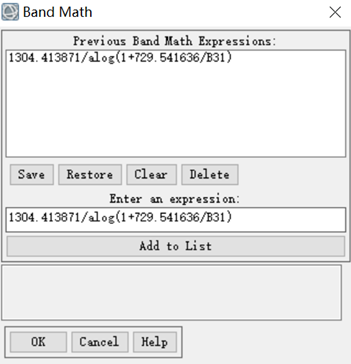地表比辐射率计算

NDVI计算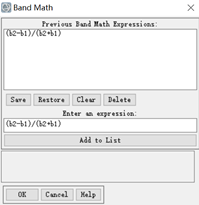$P_v$  计算
$P_v=b1 gt 0.7*1+b1 lt 0.05*0+b1 ge 0.05 and b1 le 0.7*((b1-0.05)/(0.7-0.05))$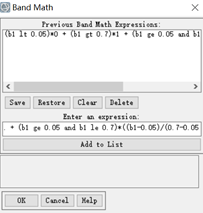$d_\varepsilon$ 计算
$d_\varepsilon=\left(b_1\ eq\ 0\ or\ b_1\ eq\ 1\right)\ast0+\left(b_1\ ge\ 0\ and\ b_1\ le\ 0.5\right)\ast0.003796\ast b_1+\left(b_1\ ge\ 0.5\ and\ b_1\ le\ 1\right)\ast0.0037968\ast1-b1+b1eq 0.5*0.00189$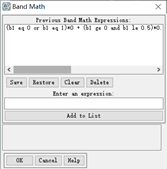$\varepsilon_i$ 计算
$b1*(0.92762+0.07033*b1)*0.98672+(1-b1)*(0.99782+0.08362*b1)*0.96767+b2$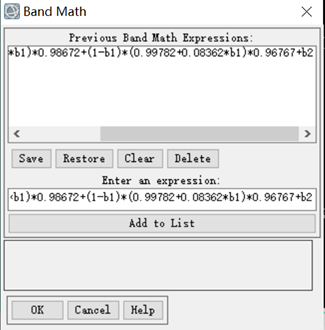大气透过率计算

W 水汽含量计算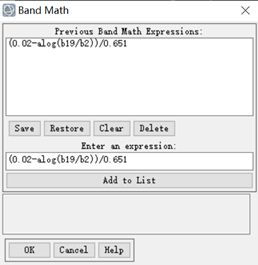$T31-T32$ 大气透过率计算
$\tau_{31}=5.72-4.69e^{(x/42.05)}$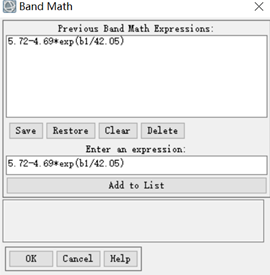$\tau_{32}=-1.25-2.28e^{(-x/12.44)}$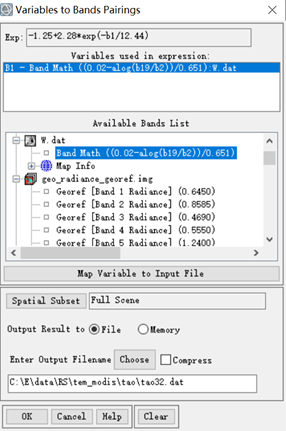计算参数 $C_i D_i$

$C_i$ 计算

$C_{31}=\varepsilon_{31}\times\tau_{31}$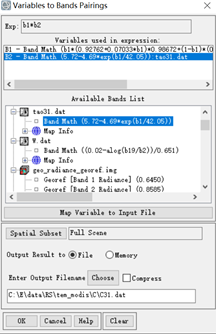$C_{32}=\varepsilon_{32}\times\tau_{32}$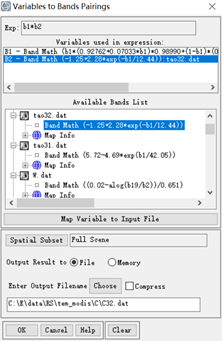$D_i$  计算
$D_i=1-τi1+1-εiτi=(1-τi)(2-Ci)$
$D_{31}=(1-\tau_{31})(2-C_{31})$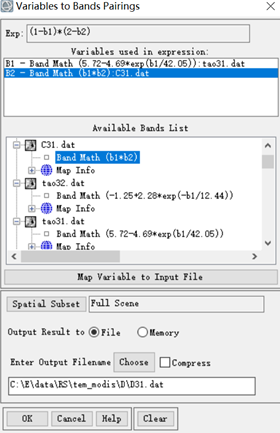$D_{32}=(1-\tau_{32})(2-C_{32})$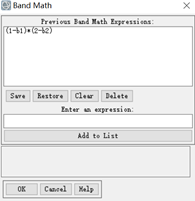计算参数 $A_{0\ }\ \ A_1\ \ A_2$

计算 $A_0$

$A_0=\frac{a31D32(1-C31-D31)}{D32C31-D31C32}-\frac{a32D31（1-C32-D32）}{D32C31-D31C32}$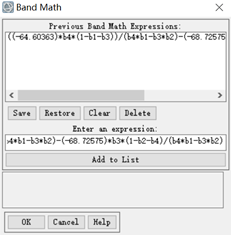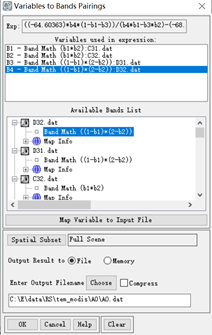计算 $A_1$

$A_1=1+\frac{D_{31}}{D_{32}C_{31}-D_{31}C_{32}}+\frac{b_{31}D_{32}(1-C_{31}-D_{31})}{D_{32}C_{31}-D_{31}C_{32}}$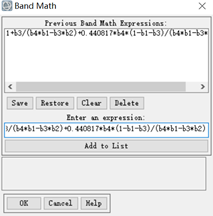计算 $A_2$

$A_2=\frac{D_{31}}{D_{32}C_{31}-D_{31}C_{32}}+\frac{{b_{32}D}_{31}(1-C_{31}-D_{32})}{D_{32}C_{31}-D_{31}C_{32}}$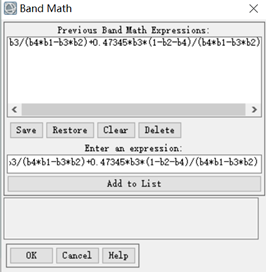计算地表温度
$T_s=A_0+A_1T_{31}-A_2T_{32}$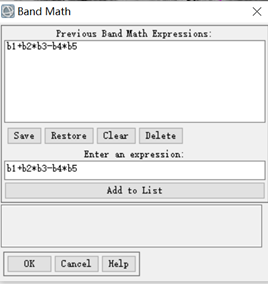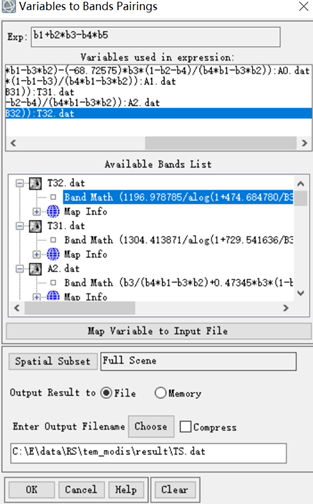反演结果

由于中间过程步骤有一步单位需要换算，所以计算结果还需要 $\div10$
ArcGIS 制图
在ARCGIS 中对反演结果进行可视化表达，加上等温线，显得更加直观。根据反演结果，发现北京市西南部温度比北部温度高，与现实情况，北京北部为怀柔、密云区，多山体和水域（密云水库）等，而北京市建成区在南部，所以呈现出这样的温度特征。说明反演结果较为准确。展开全文• 基于Landsat ETM+影像的干旱半干旱地区地表温度反演研究与分析，许民，刘勇，陆地表面温度是地-气相互作用及其能量相互交换的综合反映，研究区甘肃武威地处干旱、半干旱地区，其地表温度（LST）与下垫面覆盖�
• 地表温度反演程序使用手册，更加方便了大家对地表温度的反演，希望对相关领域的人有助
• 通过单窗算法与单通道算法来实现地表温度反演，基于ENVI进行的IDL二次开发，支持.dat格式的遥感影像。大气平均温度通过气象网站得到研究区的历年数据，大气水汽含量由经验公式所确定。两种方法所反演的地表温度相差...
• 主要详细记录了地表温度反演的操作，土地覆盖分类过程较粗，后处理部分还有待补充和完善。
• 前天刚下的Envi，高强度学习了两天摸索出了点门道，不一定准确。本文谨作为个人记录，如有问题欢迎指正。...地表温度反演操作总结 技术流程 1.数据预处理 1.1数据获取 首先在地理空间数据云下载lanenvi 单窗算法
• 这个用的是MOD02数据，对地表温度反演需要用到的几个波段分别是波段2、19、31、32。注意后面并没有做重投影，hdf数据需要build GLT，如果有需要可以看上一篇文章，可以自行加入，如果数据多，可以自己加for循环。 ...经验分享
• 地表温度作为地球环境分析的重要指标，而遥感技术作为现代重要的对地观测手段，使得基于遥感图像的地表温度反演的研究越来越多。主要的地表温度反演方法有：大气校正法，单窗算法，单通道法等等。本文介绍用辐射传输...math image class 算法...